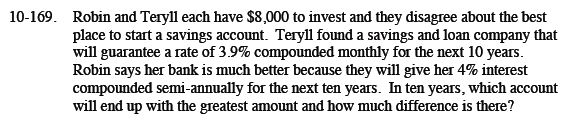Home > CCA2 > Chapter Ch10 > Lesson 10.3.2 > Problem10-169

10-169.

Robin and Teryll each have \$8,000 to invest and they disagree about the best place to start a savings account. Teryll found a savings and loan company that will guarantee a rate of 3.9% compounded monthly for the next 10 years. Robin says her bank is much better because they will give her 4% interest compounded semi-annually for the next ten years. In ten years, which account will end up with the greatest amount and how much difference is there? Homework Help ✎Use the formula for compound interest given in the Math Notes box in this lesson.

$A=8000\left(1+\frac{0.039}{12}\right)^{(12(10))}$

$A=8000\left(1+\frac{0.04}{2}\right)^{(2(10))}$